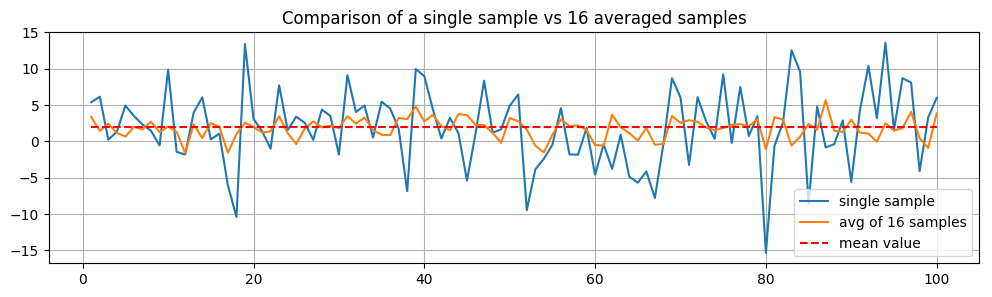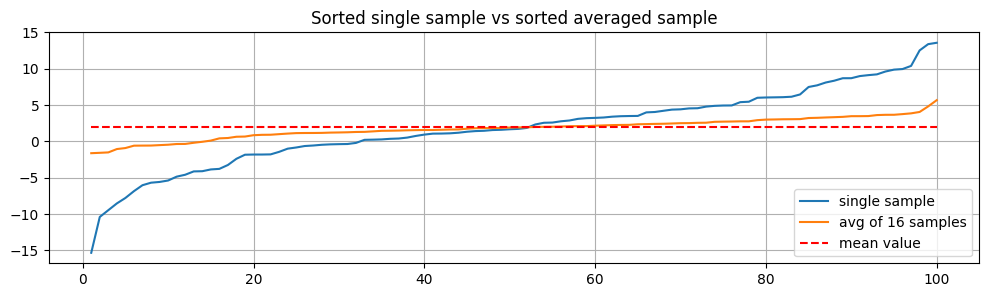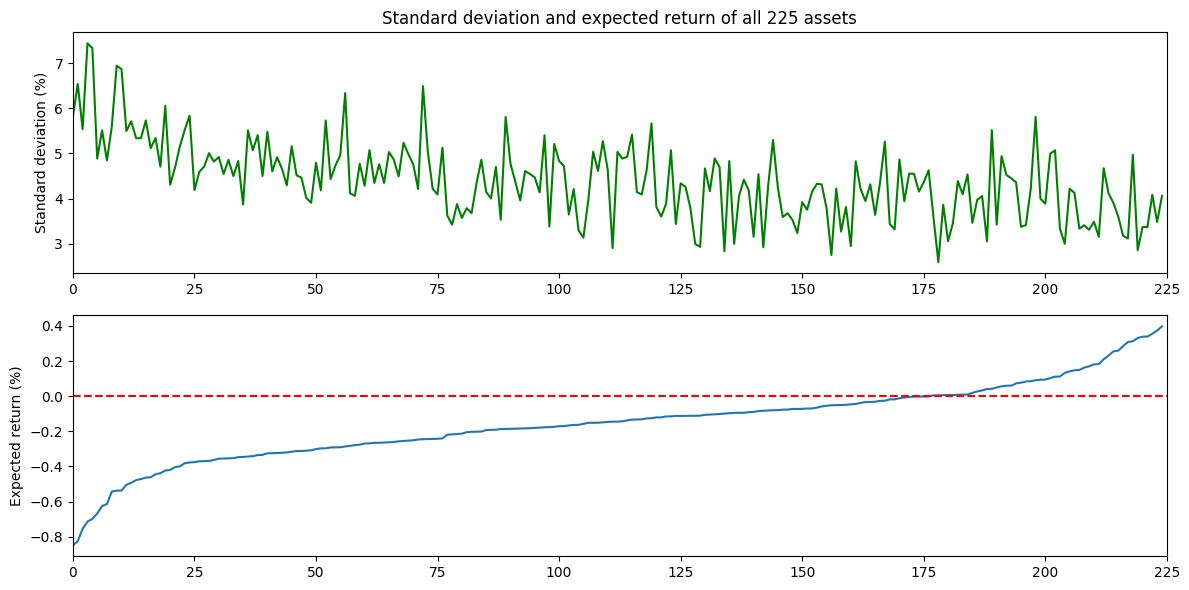# Warm-up example¶

### Averaging uncorrelated random variables reduces variance!¶

In :
srand(0)
X = 2 + 5*randn(100,16)

using PyPlot

figure(figsize=[12,3])
plot(1:100, X[:,1], 1:100, mean(X,2), 1:100, 2*ones(100), "r--")
legend(["single sample","avg of 16 samples","mean value"],loc="lower right")
title("Comparison of a single sample vs 16 averaged samples")
grid()

figure(figsize=[12,3])
plot(1:100, sort(X[:,1]), 1:100, sort(mean(X,2)[:]), 1:100, 2*ones(100), "r--")
legend(["single sample","avg of 16 samples","mean value"],loc="lower right")
title("Sorted single sample vs sorted averaged sample")
grid()
;# Portfolio optimization example¶

In :
μ = 100*readcsv("folio_mean.csv")[:]   # Expected return (in percent)
Σ = 10000*readcsv("folio_cov.csv")     # Expected variance (in percent squared)

ix = sortperm(μ);   # sort the assets by expected return

using PyPlot
fig = figure(figsize=(12,6))

subplot(211)
xlim(0,225)
plot(sqrt.(diag(Σ))[ix],"g")
ylabel("Standard deviation (%)")
title("Standard deviation and expected return of all 225 assets")
tight_layout()

subplot(212)
xlim(0,225)
plot(μ[ix])
plot([0,225],[0,0],"r--")
ylabel("Expected return (%)")
tight_layout()
#savefig("folio1_vals.pdf")
;In :
# plot correlation matrix (shows which assets are correlated with which other assets)
corr = diagm(diag(Σ).^(-1/2))*Σ*diagm(diag(Σ).^(-1/2))
imshow(corr[ix,ix]);colorbar();axis("image")
title("Correlation matrix for 225 assets")
tight_layout()
#savefig("folio2_cov.pdf")